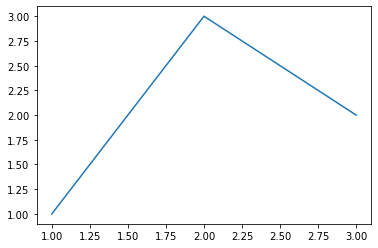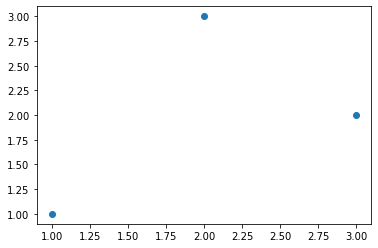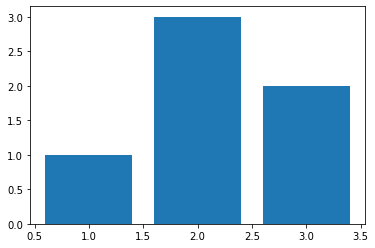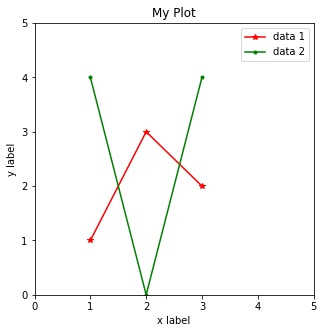### Agenda¶

1. Installation

2. Basics

3. Iterables

4. Numpy (for math and matrix operations)

5. Matplotlib (for plotting)

6. Q&A

In :
# Note: This tutorial is based on Python 3.8
#       but it should apply to all Python 3.X versions
# Please note that this tutorial is NOT exhaustive
# We try to cover everything you need for class assignments
# but you should also navigate external resources
#
# More tutorials:
# https://cs231n.github.io/python-numpy-tutorial/#numpy
# https://numpy.org/doc/stable/user/quickstart.html
# https://matplotlib.org/gallery/index.html
# https://www.w3schools.com/python/


### 1. Installation¶

#### Anaconda for environment management¶

https://www.anaconda.com/

#### Package installation using conda/pip¶

Live demo

Spyder (in-built in Anaconda)
Pycharm (the most popular choice, compatible with Anaconda)

### 2. Basics¶

In :
# input and output
name = input()
print("hello," + name)

224N
hello,224N

In :
# print multiple variables separated by a space
print("hello", name, 1, 3.0, True)

hello 224N 1 3.0 True

In :
# line comment
"""
block
"""

Out:
'\nblock \ncomments\n'
In :
# variables don't need explicit declaration
var = "hello" # string
var = 10.0    # float
var = 10      # int
var = True    # boolean
var = [1,2,3] # pointer to list
var = None    # empty pointer

In :
# type conversions
var = 10
print(int(var))
print(str(var))
print(float(var))

10
10
10.0

In :
# basic math operations
var = 10
print("var + 4 =", 10 + 4)
print("var - 4 =", 10 - 4)
print("var * 4 =", 10 * 4)
print("var ^ 4=", 10 ** 4)
print("int(var) / 4 =", 10//4)   # / for float division
print("float(var) / 4 =", 10/4)  # // for int division
# All compound assignment operators available
# including += -= *= **= /= //=
# pre/post in/decrementers not available (++ --)

var + 4 = 14
var - 4 = 6
var * 4 = 40
var ^ 4= 10000
int(var) / 4 = 2
float(var) / 4 = 2.5

In :
# basic boolean operations include "and", "or", "not"
print("not True is", not True)
print("True and False is", True and False)
print("True or False is", True or False)

not True is False
True and False is False
True or False is True

In :
# String operations
# '' and "" are equivalent
s = "String"

# basics
print(len(s)) # get length
print(s)   # get character at index
print(s[1:3]) # get substring
print("This is a " + s + "!")  # string concatenation

# handy tools
print(s.lower()) # convert to lower case
print(s*4) # repeat string
print("ring" in s) # pattern searching
print(s.index("ring")) # get index of pattern

# slice by delimiter
print("I am a sentence".split(" "))
# concatenate a list of strings using a delimiter
print(" ".join(['a','b','c']))

# formatting variables
print("Formatting a string like %.2f"%(0.12345))
print(f"Or like {s}!")

6
S
tr
This is a String!
string
StringStringStringString
True
2
['I', 'am', 'a', 'sentence']
a b c
Formatting a string like 0.12
Or like String!

In [ ]:
# control flows
# NOTE: No parentheses or curly braces
#       Indentation is used to identify code blocks
#       So never ever mix spaces with tabs
for i in range(0,5):
for j in range(i, 5):
print("inner loop")
print("outer loop")

In :
# if-else
var = 10
if var > 10:
print(">")
elif var == 10:
print("=")
else:
print("<")

=

In :
# use "if" to check null pointer or empty arrays
var = None
if var:
print(var)
var = []
if var:
print(var)
var = "object"
if var:
print(var)

object

In :
# while-loop
var = 5
while var > 0:
print(var)
var -=1

5
4
3
2
1

In :
# for-loop
for i in range(3):  # prints 0 1 2
print(i)
print("-------")
# range (start-inclusive, stop-exclusive, step)
for i in range(2, -3, -1):
print(i)

0
1
2
-------
2
1
0
-1
-2

In :
# define function
def func(a, b):
return a + b
func(1,3)

Out:
4
In :
# use default parameters and pass values by parameter name
def rangeCheck(a, min_val = 0, max_val=10):
return min_val < a < max_val    # syntactic sugar
rangeCheck(5, max_val=4)

Out:
False
In :
# define class
class Foo:

# optinal constructor
def __init__(self, x):
# first parameter "self" for instance reference, like "this" in JAVA
self.x = x

# instance method
def printX(self): # instance reference is required for all function parameters
print(self.x)

# class methods, most likely you will never need this
@classmethod
def printHello(self):
print("hello")

obj = Foo(6)
obj.printX()

6

In :
# class inheritance - inherits variables and methods
class Bar(Foo):
pass
obj = Bar(3)
obj.printX()

3


### 3. Iterables¶

In :
from collections import Counter, defaultdict
alist = list()  # linear, size not fixed, not hashable
atuple = tuple() # linear, fixed size, hashable
adict = dict()  # hash table, not hashable, stores (key,value) pairs
aset = set()    # hash table, like dict but only stores keys
acopy = alist.copy() # shallow copy
print(len(alist)) # gets size of any iterable type

0

In :
"""
List: not hashable (i.e. can't use as dictionary key)
dynamic size
allows duplicates and inconsistent element types
dynamic array implementation
"""
# list creation
alist = [] # empty list, equivalent to list()
alist = [1,2,3,4,5] # initialized list

# list indexing
print("----------------")
print(alist) # get first element (at index 0)
print(alist[-1]) # get last element (at index len-1)
print(alist[3:]) # get elements starting from index 3 (inclusive)
print(alist[:3]) # get elements stopping at index 3 (exclusive)
print(alist[2:4]) # get elements within index range [2,4)
print(alist[6:]) # prints nothing because index is out of range
print(alist[::-1]) # returns a reversed list
print("----------------")

# list modification
alist.append("new item") # insert at end
alist.insert(0, "new item") # insert at index 0
alist.extend([2,3,4]) # concatenate lists
# above line is equivalent to alist += [2,3,4]
alist.index("new item") # search by content
alist.remove("new item") # remove by content
alist.pop(0) # remove by index

# list traversal
for ele in alist:
print(ele)
print("----------------")

# or traverse with index
for i, ele in enumerate(alist):
print(i, ele)

----------------
1
5
[4, 5]
[1, 2, 3]
[3, 4]
[]
[5, 4, 3, 2, 1]
----------------
2
3
4
5
new item
2
3
4
----------------
0 2
1 3
2 4
3 5
4 new item
5 2
6 3
7 4

In :
"""
Tuple: hashable (i.e. can use as dictionary key)
fixed size (no insertion or deletion)
"""
# it does not make sense to create empty tuples
atuple = (1,2,3,4,5)
# or you can cast other iterables to tuple
atuple = tuple([1,2,3])

# indexing and traversal are same as list

In :
"""
"""
from collections import namedtuple
Point = namedtuple('Point', 'x y')
pt1 = Point(1.0, 5.0)
pt2 = Point(2.5, 1.5)
print(pt1.x, pt1.y)

1.0 5.0

In :
"""
Dict: not hashable
dynamic size
no duplicates allowed
hash table implementation which is fast for searching
"""
# dict creation
adict = {} # empty dict, equivalent to dict()
adict = {'a':1, 'b':2, 'c':3} # with initial values

# get value paired with key
# NOTE: accessing keys not in the dictionary leads to exception

print("----------------")
# add or modify dictionary entries

# get all keys in dictionary
print("----------------")

# traverse keys only
print("----------------")

# or traverse key-value pairs together
print(key, value)

3
----------------
dict_keys(['a', 'b', 'c', 'd'])
----------------
a 1
b 2
c 10
d 11
----------------
a 1
b 2
c 10
d 11

In :
"""
Special dictionaries
"""
# set is a dictionary without values
aset = set()

# deduplication short-cut using set
alist = [1,2,3,3,3,4,3]
deduplicated_list = list(set(alist))
print(deduplicated_list)

# default_dictionary returns a value computed from a default function
#     for non-existent entries
from collections import defaultdict

# counter is a dictionary with default value of 0
#     and provides handy iterable counting tools
from collections import Counter

# initialize counter from iterable
counter1 = Counter('letters to be counted')
print(counter1)

# initialize and modify empty counter
counter2 = Counter()
counter2['t'] = 10
counter2['t'] += 1
print(counter2)

# merge counters
counter3 = counter1 + counter2 # add counts
print(counter3)

[1, 2, 3, 4]
feline
unknown
Counter({'e': 4, 't': 4, ' ': 3, 'o': 2, 'l': 1, 'r': 1, 's': 1, 'b': 1, 'c': 1, 'u': 1, 'n': 1, 'd': 1})
Counter({'t': 11})
Counter({'t': 15, 'e': 4, ' ': 3, 'o': 2, 'l': 1, 'r': 1, 's': 1, 'b': 1, 'c': 1, 'u': 1, 'n': 1, 'd': 1})

In :
# Syntax sugar: one-line control flow + list operation
sent = ["i am good", "a beautiful day", "HELLO FRIEND"]
a = [s.lower().split() for s in sent]
print(a)
b = [s.lower().split() for s in sent if len(s) >10]
print(b)

[['i', 'am', 'good'], ['a', 'beautiful', 'day'], ['hello', 'friend']]
[['a', 'beautiful', 'day'], ['hello', 'friend']]

In :
# Syntax sugar: * operator for repeating iterable elements
print("-" * 10)
print( * 10)
print(['a'] * 10)
# Note, this is repeating by value
# So the elements in list cannot be objects
# To build a double list
# DO
doublelist = [[] for _ in range(10)] # each inner list is a new instance
doublelist.append(1)
print(doublelist)
# DON'T
doublelist = [[]] * 10 # each inner list is an alias of the same instance
doublelist.append(1)
print(doublelist)

----------
[1, 1, 1, 1, 1, 1, 1, 1, 1, 1]
['a', 'a', 'a', 'a', 'a', 'a', 'a', 'a', 'a', 'a']
[, [], [], [], [], [], [], [], [], []]
[, , , , , , , , , ]


### 4. Numpy¶

Very powerful python tool for handling matrices and higher dimensional arrays

In :
import numpy as np

In :
# create arrays from existing lists
a = np.array([[1,2],[3,4],[5,6]])
print(a)
print(a.shape)
print('-------')
# create all-zero/one arrays
b = np.ones([3,4]) # or np.zeros(shape)
print(b)
print(b.shape)
print('-------')
# create identity matrix
c = np.eye(5)
print(c)
print(c.shape)

[[1 2]
[3 4]
[5 6]]
(3, 2)
-------
[[1. 1. 1. 1.]
[1. 1. 1. 1.]
[1. 1. 1. 1.]]
(3, 4)
-------
[[1. 0. 0. 0. 0.]
[0. 1. 0. 0. 0.]
[0. 0. 1. 0. 0.]
[0. 0. 0. 1. 0.]
[0. 0. 0. 0. 1.]]
(5, 5)

In :
# reshaping arrays
a = np.arange(8)
b = a.reshape((4,2))     # shape [4,2]
c = a.reshape((2,2,-1))  # shape [2,2,2] -- -1 for auto-fill
d = c.flatten()          # shape [8,]
e = np.expand_dims(a, 0) # shape[1,8]    -- add new axis on 0th dimension
f = np.expand_dims(a, 1) # shape[8,1]    -- add new axis on 1st dimension
g = e.squeeze()          # shape[8, ]    -- remove all unnecessary dimensions
print(a)
print(b)

[0 1 2 3 4 5 6 7]
[[0 1]
[2 3]
[4 5]
[6 7]]

In :
# concatenating arrays
a = np.zeros((4,3))
b = np.zeros((4,3))
c = np.concatenate([a,b], axis=0)
d = np.concatenate([a,b], axis=1)
print(c.shape)
print(d.shape)

(8, 3)
(4, 6)

In :
# access array slices by index
a = np.zeros([10,10])
a[:3] = 1          # modify the first three rows together
a[:, :3] = 2       # modify the first three columns together
a[:3,:3] = 3       # modify the first three columns of the first three rows
rows = [4,6,7]
cols = [9,3,5]
a[rows, cols] = 4  # modify arbitrary positions
print(a)

[[3. 3. 3. 1. 1. 1. 1. 1. 1. 1.]
[3. 3. 3. 1. 1. 1. 1. 1. 1. 1.]
[3. 3. 3. 1. 1. 1. 1. 1. 1. 1.]
[2. 2. 2. 0. 0. 0. 0. 0. 0. 0.]
[2. 2. 2. 0. 0. 0. 0. 0. 0. 4.]
[2. 2. 2. 0. 0. 0. 0. 0. 0. 0.]
[2. 2. 2. 4. 0. 0. 0. 0. 0. 0.]
[2. 2. 2. 0. 0. 4. 0. 0. 0. 0.]
[2. 2. 2. 0. 0. 0. 0. 0. 0. 0.]
[2. 2. 2. 0. 0. 0. 0. 0. 0. 0.]]

In :
# transposition
transposed = c.T
# pinv is pseudo inversion for stability
inversed = np.linalg.pinv(c)
# l2 norm by default, read documentation for more options
norm = np.linalg.norm(c)
# the optional axis parameter
sum_all = np.sum(c) # sum all values
sum_by_row = np.sum(c, axis=0) # sum along the first axis

In :
# matrix multiplication
a = np.ones((4,3))
b = np.ones((3,2))
print(a @ b)
c = np.array([1,2,3]).reshape(3,1)
print(b+c) # automatic repeating along axis, handy in batch operations

[[3. 3.]
[3. 3.]
[3. 3.]
[3. 3.]]
[[2. 2.]
[3. 3.]
[4. 4.]]

In :
# dot product
c = np.array([1,2])
d = np.array([3,4])
print(np.dot(c,d))

11

In :
# speed test: numpy vs list
a = np.ones((100,100))
b = np.ones((100,100))

def matrix_multiplication(X, Y):
result = [*len(Y) for _ in range(len(X))]
for i in range(len(X)):
for j in range(len(Y)):
for k in range(len(Y)):
result[i][j] += X[i][k] * Y[k][j]
return result

import time

# run numpy matrix multiplication for 10 times
start = time.time()
for _ in range(10):
a @ b
end = time.time()
print("numpy spends {} seconds".format(end-start))

# run list matrix multiplication for 10 times
start = time.time()
for _ in range(10):
matrix_multiplication(a,b)
end = time.time()
print("list operation spends {} seconds".format(end-start))

# the difference gets more significant as matrices grow in size!

numpy spends 0.002250194549560547 seconds
list operation spends 7.666390657424927 seconds

In :
# element-wise operations, for examples
np.log(a)
np.exp(a)
np.sin(a)
# operation with scalar is interpreted as element-wise
a * 3

Out:
array([[3., 3., 3., ..., 3., 3., 3.],
[3., 3., 3., ..., 3., 3., 3.],
[3., 3., 3., ..., 3., 3., 3.],
...,
[3., 3., 3., ..., 3., 3., 3.],
[3., 3., 3., ..., 3., 3., 3.],
[3., 3., 3., ..., 3., 3., 3.]])

### 5. Matplotlib¶

Powerful tool for visualization
Many tutorials online. We only go over the basics here

In :
import matplotlib.pyplot as plt

In :
# line plot
x = [1,2,3]
y = [1,3,2]
plt.plot(x,y)

Out:
[<matplotlib.lines.Line2D at 0x7f667c9959a0>]In :
# scatter plot
plt.scatter(x,y)

Out:
<matplotlib.collections.PathCollection at 0x7f667c9194c0>In :
# bar plots
plt.bar(x,y)

Out:
<BarContainer object of 3 artists>In :
# plot configurations
x = [1,2,3]
y1 = [1,3,2]
y2 = [4,0,4]

# set figure size
plt.figure(figsize=(5,5))

# set axes
plt.xlim(0,5)
plt.ylim(0,5)
plt.xlabel("x label")
plt.ylabel("y label")

plt.title("My Plot")

# set line features
plt.plot(x,y1, color="red", label="data 1", marker="*")
plt.plot(x,y2, color="green", label="data 2", marker=".")

# show legends
plt.legend()

Out:
<matplotlib.legend.Legend at 0x7f667c8dbca0>### Q&A¶

In [ ]: